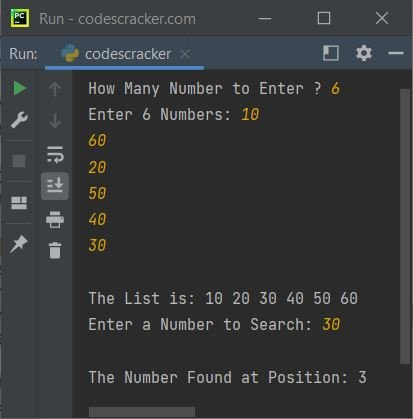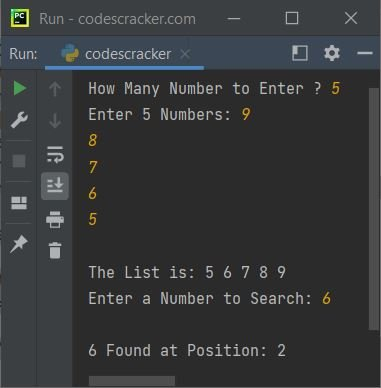# Binary Search Program in Python

In this article, you will learn and get code to implement binary search in a Python program. Here is a list of programs for binary search:

• Binary Search using a List with Ordered Inputs
• Binary Search with Random Inputs of a Given Size
• Binary Search using for Loop

Before going through these programs, if you're not aware of the topic, then refer to the binary search logic and algorithm to get everything required.

## Binary search using lists

This program searches a number entered by the user using the binary search technique. The program receives 10 numbers from the user and then a number to search using binary search.

```nums = []
print("Enter 10 Numbers (in ascending order):")
for i in range(10):
nums.insert(i, int(input()))
print("Enter a Number to Search:")
search = int(input())
first = 0
last = 9
middle = (first+last)/2
middle = int(middle)
while first <= last:
if nums[middle]<search:
first = middle+1
elif nums[middle]==search:
print("The Number Found at Position:")
print(middle+1)
break
else:
last = middle-1
middle = (first+last)/2
middle = int(middle)
if first>last:

Here is its sample run:Now supply any 10 numbers, say 10, 20, 30, 40, 50, 60, 70, 80, 90, and 100, and then a number, say 60, to search. Here is the sample run with exactly these inputs:The range() function returns a sequence of values. Its limit is decided by its argument. For example:

```for i in range(10):
print(i)```

prints the value of i ten times, starting from 0 to 9. Therefore, 0, 1, 2, 3, 4, 5, 6, 7, 8, and 9 get printed on the output. Each number is printed on a new line. Therefore, the following code (from the above program):

`for i in range(10):`

is used to execute the following statements:

`nums.insert(i, int(input()))`

ten times with a value of i from 0 to 9.

Note: The insert() function is used to insert an element into the list (nums[] here). Therefore, the user is allowed to enter the value ten times. And all the values get stored in nums[] in the following way:

• The first number entered by the user gets stored in nums[i] or nums.
• The second number gets stored in nums.
• The third number gets stored in nums.
• and so on up to
• The tenth number gets stored in nums.

Note: In above program, the position is printed with a middle+1 value because indexing starts with 0. Therefore, the 0th index's value is referred as the first number.

### Binary Search with Random User Inputs of a Given Sizee

This program is similar to the previous one with two extra features. The first one is that this program allows the user to define the size of the list. The second one is that this program doesn't care about the order in which the user enters the data. We've implemented the code to sort the list before applying the binary search technique to search a number.

```nums = []
print(end="How Many Number to Enter ? ")
tot = int(input())
print(end="Enter " + str(tot) + " Numbers: ")
for i in range(tot):
nums.insert(i, int(input()))

for i in range(tot-1):
for j in range(tot-i-1):
if nums[j]>nums[j+1]:
temp = nums[j]
nums[j] = nums[j+1]
nums[j+1] = temp

print(end="\nThe List is: ")
for i in range(tot):
print(end=str(nums[i]) + " ")

print(end="\nEnter a Number to Search: ")
search = int(input())
first = 0
last = tot-1
middle = int((first+last)/2)
while first <= last:
if nums[middle]<search:
first = middle+1
elif nums[middle]==search:
print("\nThe Number Found at Position: " + str(middle+1))
break
else:
last = middle-1
middle = int((first+last)/2)
if first>last:

Here is a sample run with the following user inputs:

• 6 as the size of numbers
• 10, 60, 20, 50, 40, and 30 as 6 numbers
• 30 as the number to search

Based on exactly these inputs, here is the sample run:Note: The position of the entered number, say 30, gets calculated based on the sorted list, not on the original (unordered) list. because the binary search technique works on a sorted list.

## Binary Search using for Loop

To create the same program as the previous one, but using a "for" loop. Then the main thing to change is:

`while first <= last:`

with this:

`for first in range(last+1):`

Here is the complete program for binary search using the "for" loop in Python:

```nums = []
chk = 0
print(end="How Many Number to Enter ? ")
tot = int(input())
print(end="Enter " + str(tot) + " Numbers: ")
for i in range(tot):
nums.insert(i, int(input()))

for i in range(tot-1):
for j in range(tot-i-1):
if nums[j]>nums[j+1]:
temp = nums[j]
nums[j] = nums[j+1]
nums[j+1] = temp

print(end="\nThe List is: ")
for i in range(tot):
print(end=str(nums[i]) + " ")

print(end="\nEnter a Number to Search: ")
search = int(input())
first = 0
last = tot-1
middle = int((first+last)/2)
for first in range(last+1):
if nums[middle]<search:
first = middle+1
elif nums[middle]==search:
print("\n" + str(search) + " Found at Position: " + str(middle+1))
chk = 1
break
else:
last = middle-1
middle = int((first+last)/2)
if chk!=1:

Here is its sample run:Python Online Test

« Previous Program Next Program »